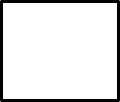1
visibility

Determine the number that makes the equation true.+ 2 = 41 – 8

• A

33

• B

28

• C

31

• D

30

The correct answer is 31.

Solution:
Consider the given equation.+ 2 = 41 – 8

To determine the missing number, first, we need to find: 41 – 8.

Which gives:
41 - 8 = 33

Then,+ 2 = 41 – 8+ 2 = 33

Next, to find the missing number we find: 33 – 2.

Which gives:
33 – 2 = 31

So,
31 + 2 = 41 – 8

That is, number 31 makes the equation true.